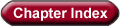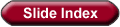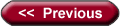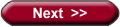## Q - Notation:

For a given function g (n) :
Q (g(n)) = { f (n) : \$ constants c1, c2, n0  │  0  ú  c1 g (n)   ú  f (n) ú  c2 g (n)  " n   │  n0

This means: At some point F (n) is sandwiched between c1 g (n) and c2 g (n) :from Cormen figure 2.1

Q ( g (n) ) is a set of functions, yet we write " f (n) = Q ( g (n) )" to mean that f (n) Q ( g (n) ) We use set notation because of sandwiching for  n   │    f (n) is equal to g (n) to within a constant factor, therefore g (n) is an asymptotically tight bound for f (n).

Note: The definition requires all f (n) Q ( g (n) ) to be asymptotically non-negative. This means that g (n) must be as well.

Example Problem:

Show that f (n) = n2/2 - 3n  ╬  Q ( n2 )  -- we must find n0, c1,c2 for  this definition that fit the equation:

c1 n2   ú  n2/2 - 3n  ú  c2 n2    " n   │  n0

c1   ú  1/2 - 3/n  ú  cby dividing by  n2

If n │  1 then 1/2 - 3/n ú  1/2  by making c2 equal to 1/2

1/2 - 3/n  │  1/14 when n  7   ( 1/2 - 3/n = 0 when n = 6 )

So c1 = 1/14, c2   = 1/2,  n=  7

Note: other constants work, but we FOUND a set that does.

Consider the contradiction  6 n ╣  Q ( n2 ) Suppose this were true:

6 nú  c2 n2   " n   │  n0

6   ú  c2/n  " n   │  n0 then n  ú  c2/6

A note on condensing expressions:  f (n) = an2  + bn + c is Q ( n2 ), in fact:

P (n )    =
 d Õ i = 0 aini     =
adnd + ....+ a0  -- degree d polynomial is  Q ( nd )

(proofs are in the text book)